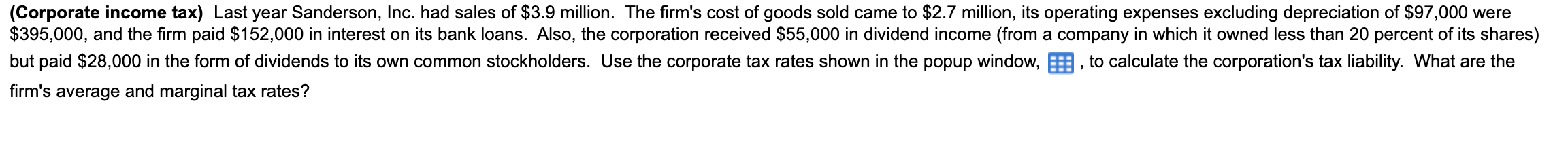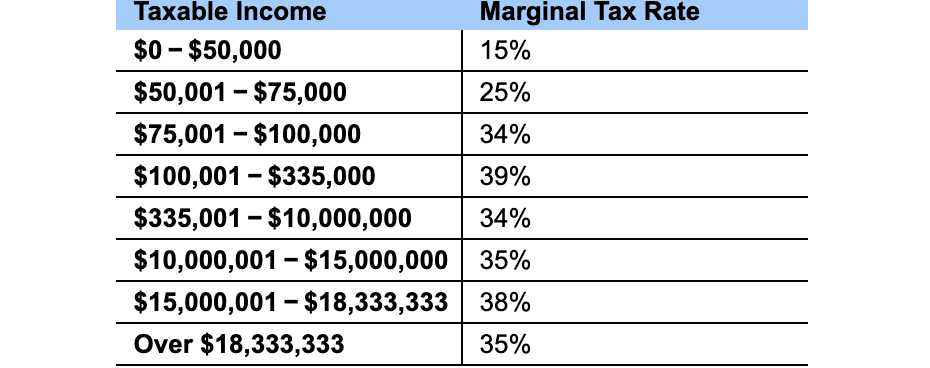# (Solved): Last year Sanderson, Inc. had sales of $3.9 million. The firm's cost of goods sold came to ... Last year? Sanderson, Inc. had sales of$3.9 million. The? firm's cost of goods sold came to ?$2.7 million, its operating expenses excluding depreciation of$97,000 were $395,000, and the firm paid$152,000 in interest on its bank loans. ? Also, the corporation received $55,000 in dividend income? (from a company in which it owned less than 20 percent of its? shares) but paid$28,000 in the form of dividends to its own common stockholders. Use the corporate tax rates shown in the popup? window to:

1. calculate the? corporation's tax liability

2. What are the? firm's average and marginal tax? rates?firm's average and marginal tax rates? \begin{tabular}{l|l} Taxable Income & Marginal Tax Rate \\ $$\ 0-\ 50,000$$ & $$15 \%$$ \\ \hline$$\ 50,001-\ 75,000$$ & $$25 \%$$ \\ \hline$$\ 75,001-\ 100,000$$ & $$34 \%$$ \\ \hline$$\ 100,001-\ 335,000$$ & $$39 \%$$ \\ \hline$$\ 335,001-\ 10,000,000$$ & $$34 \%$$ \\ \hline$$\ 10,000,001-\ 15,000,000$$ & $$35 \%$$ \\ \hline$$\ 15,000,001-\ 18,333,333$$ & $$38 \%$$ \\ \hline Over $$\ 18,333,333$$ & $$35 \%$$ \\ \hline \end{tabular}

We have an Answer from Expert